#JavaScript数字精度丢失问题总结

0
0
01. 云栖社区>
2. 博客>
3. 正文

## JavaScript数字精度丢失问题总结

1. JS 数字精度丢失的一些典型问题
2. JS 数字精度丢失的原因
3. 解决方案（一个对象+一个函数）

## 一、JS数字精度丢失的一些典型问题

1. 两个简单的浮点数相加

```0.1 + 0.2 != 0.3 // true
```

Firebug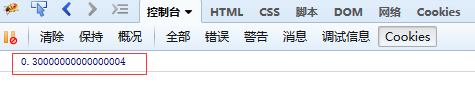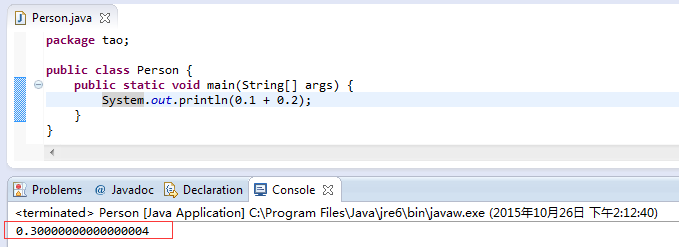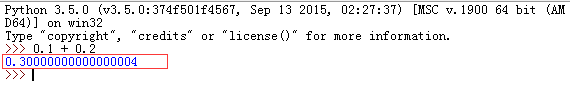2. 大整数运算

```9999999999999999 == 10000000000000001 // ？
```

Firebug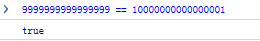16位和17位数竟然相等，没天理啊。

```var x = 9007199254740992
x + 1 == x // ？
```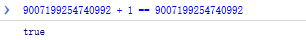3. toFixed 不会四舍五入（Chrome）

`1.335.toFixed(2) // 1.33`

Firebug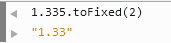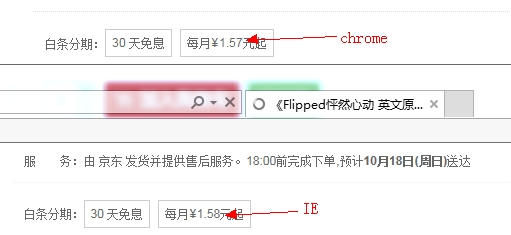## 二、JS 数字丢失精度的原因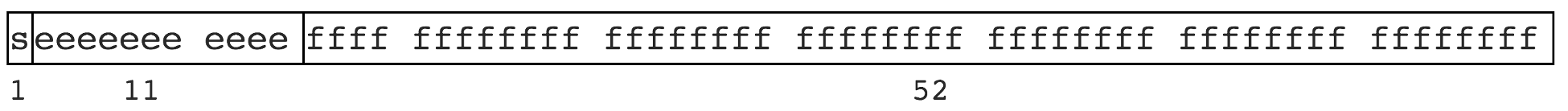• 1位用来表示符号位
• 11位用来表示指数
• 52位表示尾数

```0.1 >> 0.0001 1001 1001 1001…（1001无限循环）
0.2 >> 0.0011 0011 0011 0011…（0011无限循环）
```

```9007199254740992     >> 10000000000000...000 // 共计 53 个 0
9007199254740992 + 1 >> 10000000000000...001 // 中间 52 个 0
9007199254740992 + 2 >> 10000000000000...010 // 中间 51 个 0
```

```9007199254740992 + 1 // 丢失
9007199254740992 + 2 // 未丢失
9007199254740992 + 3 // 丢失
9007199254740992 + 4 // 未丢失
```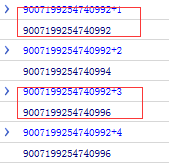## 三、解决方案

```// 0.1 + 0.2
(0.1*10 + 0.2*10) / 10 == 0.3 // true
```

```/**
* floatObj 包含加减乘除四个方法，能确保浮点数运算不丢失精度
*
* 我们知道计算机编程语言里浮点数计算会存在精度丢失问题（或称舍入误差），其根本原因是二进制和实现位数限制有些数无法有限表示
* 以下是十进制小数对应的二进制表示
*      0.1 >> 0.0001 1001 1001 1001…（1001无限循环）
*      0.2 >> 0.0011 0011 0011 0011…（0011无限循环）
* 计算机里每种数据类型的存储是一个有限宽度，比如 JavaScript 使用 64 位存储数字类型，因此超出的会舍去。舍去的部分就是精度丢失的部分。
*
* ** method **
*  add / subtract / multiply /divide
*
* ** explame **
*  0.1 + 0.2 == 0.30000000000000004 （多了 0.00000000000004）
*  0.2 + 0.4 == 0.6000000000000001  （多了 0.0000000000001）
*  19.9 * 100 == 1989.9999999999998 （少了 0.0000000000002）
*
* floatObj.multiply(19.9, 100) >> 1990
*
*/
var floatObj = function() {

/*
* 判断obj是否为一个整数
*/
function isInteger(obj) {
return Math.floor(obj) === obj
}

/*
* 将一个浮点数转成整数，返回整数和倍数。如 3.14 >> 314，倍数是 100
* @param floatNum {number} 小数
* @return {object}
*   {times:100, num: 314}
*/
function toInteger(floatNum) {
var ret = {times: 0, num: 0}
if (isInteger(floatNum)) {
ret.num = floatNum
return ret
}
var strfi  = floatNum + ''
var dotPos = strfi.indexOf('.')
var len    = strfi.substr(dotPos+1).length
var times  = Math.pow(10, len)
var intNum = parseInt(floatNum * times + 0.5, 10)
ret.times  = times
ret.num    = intNum
return ret
}

/*
* 核心方法，实现加减乘除运算，确保不丢失精度
* 思路：把小数放大为整数（乘），进行算术运算，再缩小为小数（除）
*
* @param a {number} 运算数1
* @param b {number} 运算数2
* @param digits {number} 精度，保留的小数点数，比如 2, 即保留为两位小数
*
*/
function operation(a, b, digits, op) {
var o1 = toInteger(a)
var o2 = toInteger(b)
var max = o1.times > o2.times ? o1.times : o2.times
var result = null
switch (op) {
result = o1.num + o2.num
break
case 'subtract':
result = o1.num - o2.num
break
case 'multiply':
result = o1.num * o2.num
break
case 'divide':
result = o1.num / o2.num
break
}
return result / max
}

// 加减乘除的四个接口
}
function subtract(a, b, digits) {
return operation(a, b, digits, 'subtract')
}
function multiply(a, b, digits) {
return operation(a, b, digits, 'multiply')
}
function divide(a, b, digits) {
return operation(a, b, digits, 'divide')
}

// exports
return {
subtract: subtract,
multiply: multiply,
divide: divide
}

}();
```

toFixed的修复如下

```// toFixed 修复
function toFixed(num, s) {
var times = Math.pow(10, s)
var des = num * times + 0.5
des = parseInt(des, 10) / times
return des + ''
}
```

http://0.30000000000000004.com

http://docs.oracle.com/cd/E19957-01/806-3568/ncg_goldberg.html

http://mathjs.org/
http://www.ituring.com.cn/article/73940
https://en.wikipedia.org/wiki/IEEE_floating_point